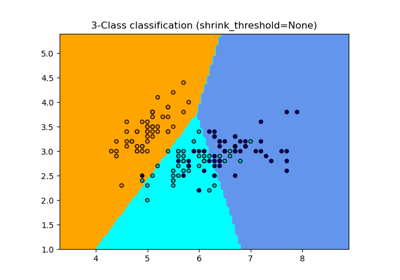# sklearn.neighbors.NearestCentroid¶

class sklearn.neighbors.NearestCentroid(metric='euclidean', *, shrink_threshold=None)[source]

Nearest centroid classifier.

Each class is represented by its centroid, with test samples classified to the class with the nearest centroid.

Read more in the User Guide.

Parameters:
metricstr or callable, default=”euclidean”

Metric to use for distance computation. Default is “minkowski”, which results in the standard Euclidean distance when p = 2. See the documentation of scipy.spatial.distance and the metrics listed in distance_metrics for valid metric values. Note that “wminkowski”, “seuclidean” and “mahalanobis” are not supported.

The centroids for the samples corresponding to each class is the point from which the sum of the distances (according to the metric) of all samples that belong to that particular class are minimized. If the "manhattan" metric is provided, this centroid is the median and for all other metrics, the centroid is now set to be the mean.

Changed in version 0.19: metric='precomputed' was deprecated and now raises an error

shrink_thresholdfloat, default=None

Threshold for shrinking centroids to remove features.

Attributes:
centroids_array-like of shape (n_classes, n_features)

Centroid of each class.

classes_array of shape (n_classes,)

The unique classes labels.

n_features_in_int

Number of features seen during fit.

New in version 0.24.

feature_names_in_ndarray of shape (n_features_in_,)

Names of features seen during fit. Defined only when X has feature names that are all strings.

New in version 1.0.

KNeighborsClassifier

Nearest neighbors classifier.

Notes

When used for text classification with tf-idf vectors, this classifier is also known as the Rocchio classifier.

References

Tibshirani, R., Hastie, T., Narasimhan, B., & Chu, G. (2002). Diagnosis of multiple cancer types by shrunken centroids of gene expression. Proceedings of the National Academy of Sciences of the United States of America, 99(10), 6567-6572. The National Academy of Sciences.

Examples

>>> from sklearn.neighbors import NearestCentroid
>>> import numpy as np
>>> X = np.array([[-1, -1], [-2, -1], [-3, -2], [1, 1], [2, 1], [3, 2]])
>>> y = np.array([1, 1, 1, 2, 2, 2])
>>> clf = NearestCentroid()
>>> clf.fit(X, y)
NearestCentroid()
>>> print(clf.predict([[-0.8, -1]]))



Methods

 fit(X, y) Fit the NearestCentroid model according to the given training data. get_params([deep]) Get parameters for this estimator. Perform classification on an array of test vectors X. score(X, y[, sample_weight]) Return the mean accuracy on the given test data and labels. set_params(**params) Set the parameters of this estimator.
fit(X, y)[source]

Fit the NearestCentroid model according to the given training data.

Parameters:
X{array-like, sparse matrix} of shape (n_samples, n_features)

Training vector, where n_samples is the number of samples and n_features is the number of features. Note that centroid shrinking cannot be used with sparse matrices.

yarray-like of shape (n_samples,)

Target values.

Returns:
selfobject

Fitted estimator.

get_params(deep=True)[source]

Get parameters for this estimator.

Parameters:
deepbool, default=True

If True, will return the parameters for this estimator and contained subobjects that are estimators.

Returns:
paramsdict

Parameter names mapped to their values.

predict(X)[source]

Perform classification on an array of test vectors X.

The predicted class C for each sample in X is returned.

Parameters:
X{array-like, sparse matrix} of shape (n_samples, n_features)

Test samples.

Returns:
Cndarray of shape (n_samples,)

The predicted classes.

Notes

If the metric constructor parameter is "precomputed", X is assumed to be the distance matrix between the data to be predicted and self.centroids_.

score(X, y, sample_weight=None)[source]

Return the mean accuracy on the given test data and labels.

In multi-label classification, this is the subset accuracy which is a harsh metric since you require for each sample that each label set be correctly predicted.

Parameters:
Xarray-like of shape (n_samples, n_features)

Test samples.

yarray-like of shape (n_samples,) or (n_samples, n_outputs)

True labels for X.

sample_weightarray-like of shape (n_samples,), default=None

Sample weights.

Returns:
scorefloat

Mean accuracy of self.predict(X) wrt. y.

set_params(**params)[source]

Set the parameters of this estimator.

The method works on simple estimators as well as on nested objects (such as Pipeline). The latter have parameters of the form <component>__<parameter> so that it’s possible to update each component of a nested object.

Parameters:
**paramsdict

Estimator parameters.

Returns:
selfestimator instance

Estimator instance.

## Examples using sklearn.neighbors.NearestCentroid¶Nearest Centroid Classification

Nearest Centroid ClassificationClassification of text documents using sparse features

Classification of text documents using sparse features